[english]
[français]

# Charles Deledalle - Teaching - Backup 2017

## UCSD - ECE285 Image and video restoration (Fall 2017)

(Matlab version)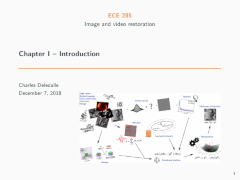Chapter 1 - Introduction (61Mb) Image sciences, image processing, image restoration, photo manipulation. Image and videos representation. Digital versus analog imagery. Quantization and sampling. Sources and models of noises in digital CCD imagery: photon, thermal and readout noises. Sources and models of blurs. Convolutions and point spread functions. Overview of other standard models, problems and tasks: salt-and-pepper and impulse noises, half toning, inpainting, super-resolution, compressed sensing, high dynamic range imagery, demosaicing. Short introduction to other types of imagery: SAR, Sonar, ultrasound, CT and MRI. Linear and ill-posed restoration problems.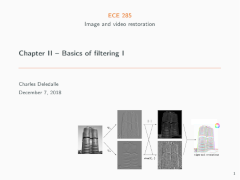Chapter 2 - Basics of filtering I: Spatial filters (25Mb) Moving averages. Finite differences and edge detectors. Gradient, Sobel and Laplacian. Linear translations invariant filters, cross-correlation and convolution. Adaptive and non-linear filters. Median filters. Morphological filters. Local versus global filters. Sigma filter. Bilateral filter. Patches and non-local means. Applications to image denoising.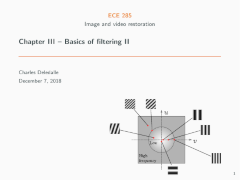Chapter 3 - Basics of filtering II: Spectral filters (17Mb) Fourier decomposition and Fourier transform. Continuous verse discrete Fourier transform. 2D Fourier transform and spectral analysis. Low-pass and high-pass filters. Convolution theorem. Image sharpening, Image resizing and sub-sampling. Aliasing, Nyquist -Shannon theorem, zero-padding, and windowing. Spectral modelization of subsampling in CT and MRI. Radon transform, k-space trajectories, and streaking artifacts.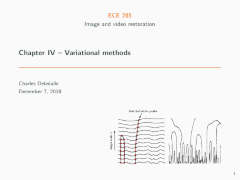Chapter 4 - Variational methods (36Mb) Heat equation. Discretization and finite difference. Explicit and implicit Euler schemes. CFL conditions. Continuous Gaussian convolution solution. Linear and non-linear scale spaces. Anisotropic diffusion. Perona-Malik and Weickert model. Variational methods. Tikhonov regularization by gradient descent. Links between variational models and diffusion models. Total-Variation regularization and ROF model. Sparsity and group sparsity. Applications to image deconvolution.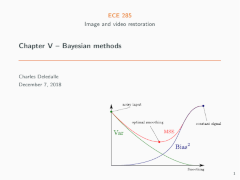Chapter 5 - Bayesian methods (58Mb) Sample mean, law of large numbers, and method of moments. Mean square error and bias-variance tradeoff. Unbiased estimation: MVUE, Cramèr-Rao-Bound, Efficiency, MLE. Linear estimation: BLUE, Gauss-Markov theorem, least-square error estimator, Moore-Penrose pseudo-inverse. Bayesian estimators: likelihood and priors, MMSE and posterior mean, MAP. Linear MMSE, applications to Wiener deconvolution, image filtering with PCA, and the non-local Bayes algorithm. Applications to image denoising.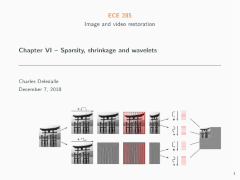Chapter 6 - Sparsity, shrinkage and wavelets (93Mb) Limits of Wiener filtering. Non-linear shrinkage functions. Limits of Fourier representation. Continuous and discrete wavelet transforms. Sparsity and shrinkage in wavelet domain. Undecimated wavelet transforms, a trous algorithm. Regularization. Sparse regression, combinatorial optimization and matching pursuit. LASSO, non-smooth optimization, and proximal minimization. Link with implcit Euler scheme. ISTA algorithm. Syntehsis versus analysis regularized models. Applications to image deconvolution.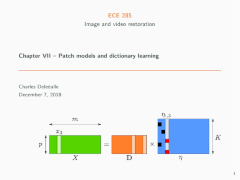Chapter 7 - Patch models and dictionary learning (34Mb) Patch models and sparse decompositions of image patches. Dictionary learning and the k-SVD algorithm. Collaborative filtering and BM3D. Non-local sparse based models. Expected patch log-likelihood. Other applications of patch models in inpainting, super-resolution and deblurring.